Dot numbers are a new notation for numbers, that make integer addition look like rational multiplication. They may be useful in primary school math education. The idea is that once you understand integers and addition, you can learn another way to look at it that sets you up to understand fractions and multiplication.

I made up dot numbers a few years ago to try to explain negative numbers to my then-four-year-old son.

### Basics

A dot number is a way of writing a number. A dot number is represented as a number of dots above a line. This is the number 3, as a dot number: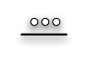A negative dot number is a number of dots below the line. This is the dot number -3: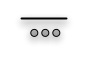.

To add two dot numbers, combine the dots above the line, and the dots below the line. This is 3 plus 2: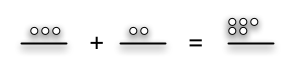This is -3 plus -2: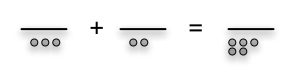And this is 3 plus -2: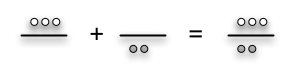### Cancelling

A dot number is in normal form if all its dots (if any) are on the same side of the line. A dot number that isn’t in normal form can be “normalizing”, or transformed into another dot number that represents the same integer and is in normal form. As long as there’s a dot above the line and a dot below the line, cross the dots out. The pairs of dots “cancel” each other.

The preceding number (3 plus -2) can be normalized.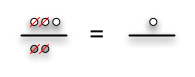To subtract a number, flip it upside down and add that. This is 3 minus 2: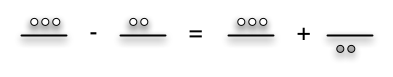### Dots and Factors

Dot numbers are interesting because they’re isomorphic to fractions. Dot numbers are to addition (and subtraction, and the unit) as fractions are to multiplication (and division, and factors).

Just as the multiplicative inverse (the reciprocal) of a fraction can be obtained by turning it upside down, the additive inverse (the negative) of a dot number is obtained by flipping it upside down. (The inverse in both cases is the “vertical inverse”).

Just as fractions are multiplied by multiplying the tops and bottoms (numerators and denominators) separately, dot numbers are added by adding their tops and bottoms.

And just as any number can be represented by multiple fractions (1/2, 2/4, 4/8) which can be normalized (“reduced”) by dividing the top and bottom by integral factors (4/8 = 4Ã—1/4Ã—2 = 4Ã—1/4Ã—2 = 1/2), so an integer (3) can be represented by multiple dot numbers (3-0, 4-1, 5-2), each of which can be reduced by subtracting units from its top and bottom (5-2 = (1+1+1+1+1)-(1+1) = (1+1+1+1+1)-(1+1+1+1+1) = (1+1+1+1+1)-(1+1+1+1+1) = 3-0).